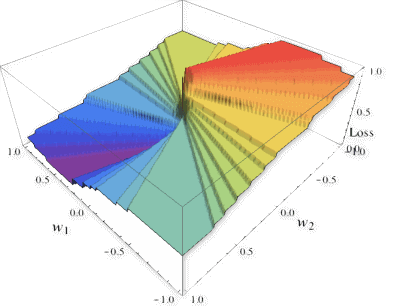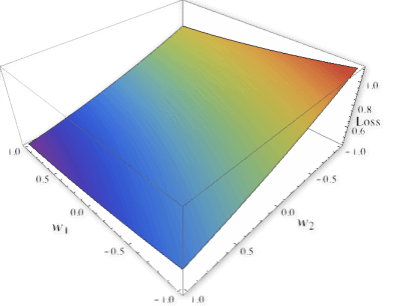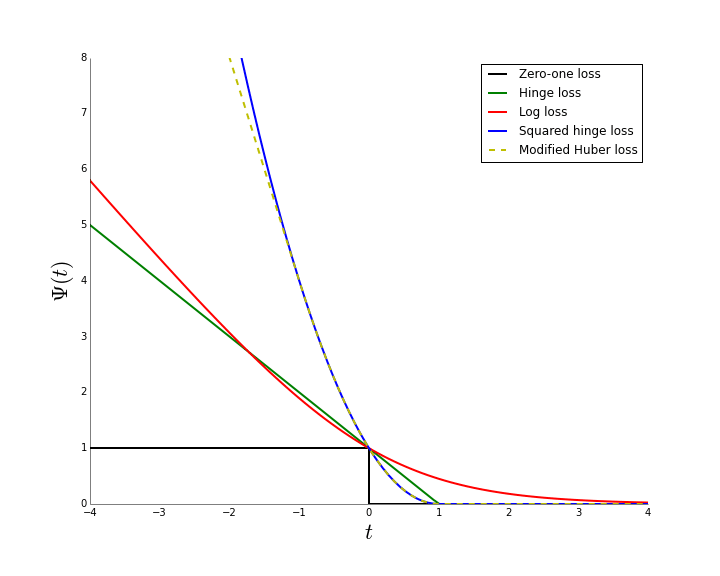#### 怎么理解surrogate loss function代理损失函数？

统计/机器学习 监督式学习 损失函数    浏览次数：15374        分享

17数据痴汉   2017-04-19 10:33

##### 2个回答
31

Surrogate loss function，中文可以译为代理损失函数。当原本的loss function不便计算的时候，我们就会考虑使用surrogate loss function。

$$l(y, \hat y)=\sum_{i=1}^m\chi(y_i\neq\hat y_i).$$

$$l(y, \hat y)=0+1+0+1+0=2.$$

$$\min_{h}\mathbb{E}_{X\times y}[l(y, h(X))].$$

$$h(X)=\begin{cases}1, ~Xw\geq 0\\ -1, ~Xw<0 \end{cases}$$SofaSofa数据科学社区DS面试题库 DS面经高代兄   2017-04-19 13:06

13

SofaSofa数据科学社区DS面试题库 DS面经Nagozi   2017-04-20 01:27相关主题